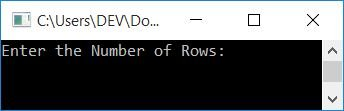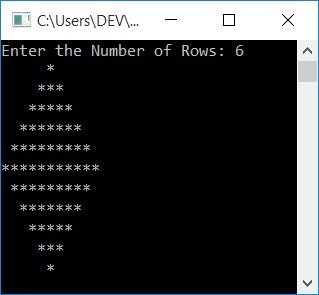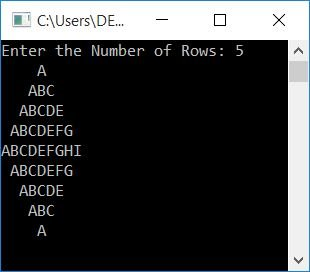# C++ program to print a diamond pattern

In this article, you will learn and get code to print a diamond pattern in C++. Here is the list of programs on the printing of diamond patterns available in this article:

## In C++, print a diamond pattern of stars

To print a diamond pattern of stars in C++ programming, you have to ask the user to enter the number of rows. Now, using the row size, print the diamond pattern as shown in the program given below:

If the user enters 6 as the row size of a diamond, then its upper-triangular part expands up to 6 lines, whereas its lower-triangular part expands up to 5 (one less than the row size) lines.

The question is, "Write a program in C++ to print a diamond pattern of stars." Here is its answer:

```#include<iostream>
using namespace std;
int main()
{
int i, j, rowNum, space;
cout<<"Enter the Number of Rows: ";
cin>>rowNum;
space = rowNum-1;
for(i=1; i<=rowNum; i++)
{
for(j=1; j<=space; j++)
cout<<" ";
space--;
for(j=1; j<=(2*i-1); j++)
cout<<"*";
cout<<endl;
}
space = 1;
for(i=1; i<=(rowNum-1); i++)
{
for(j=1; j<=space; j++)
cout<<" ";
space++;
for(j=1; j<=(2*(rowNum-i)-1); j++)
cout<<"*";
cout<<endl;
}
cout<<endl;
return 0;
}```

This program was built and runs under the Code::Blocks IDE. Here is its sample run:Now supply the input, say 6 as the number of rows, and press the ENTER key to print the diamond pattern as shown in the snapshot given below:Note that the row size in this case is the upper triangular part of the diamond pattern, not the actual row size.

There are three for loops used to create both the upper and lower triangles of a diamond. The first three for loops are created in a way that:

• The first corresponds to the rows.
• The second corresponds to spaces.
• The third corresponds to columns (the printing of stars).

And the three for loops for lower triangular part of the diamond pattern also works in a similar way.

The dry run of the following block of code:

```space = rowNum-1;
for(i=1; i<=rowNum; i++)
{
for(j=1; j<=space; j++)
cout<<" ";
space--;
for(j=1; j<=(2*i-1); j++)
cout<<"*";
cout<<endl;
}```

that prints the upper triangular part of a diamond, goes like:

• When a user enters a row size of 6, it is saved in rowNum.
• And rowNum-1, 6-1, or 5 is saved in space.
• Now, program flow starts the execution of the for loop. That is, i = 0, and the condition i<=rowNum or 0<=6 evaluates to be true.
• Therefore, program flow goes inside the loop; now j=1 and the condition j<=space or 1<=5 evaluates to be true.
• Therefore, program flow goes inside this loop and prints a single space.
• Now the value of j gets incremented. So j=2 and the condition j<=space or 2<=space or 2<=5 evaluates to true again, resulting in the printing of another space.
• In this way, there are 4 spaces that get printed, and the condition is evaluated to be false.
• After exiting this loop, decrement the value of space to print one space less from the next line or row.
• Using the third for loop, print stars.
• That is, at first execution, j=1, and the condition j<=(2*i-1) or j<=(2*1-1) or 1<=(1) or 1<=1 evaluates to be true, therefore program flow goes inside this loop and prints a star (*).
• Now the value of j gets incremented. So j=2
• And the condition j<=(2*i-1) or 2<=(2*1-1) or 2<=(1) evaluates to be false, therefore the program ends this loop.
• Using endl, begin the next thing on a new line.
• Now program flow increments the value of the outer for loop's variable, which is i. So i=2
• And the condition i<=rowNum or 2<=6 evaluates to be true again, therefore program flow goes inside the loop again, and this process continues until the condition evaluates to be false.

In this way, the star pattern of the upper diamond gets printed, and in a similar way, the lower diamond also gets printed.

## Print a diamond pattern of numbers

This is the same program as the previous one. The only difference is that instead of using stars, we've used numbers to print a diamond pattern of numbers.

```#include<iostream>
using namespace std;
int main()
{
int i, j, rowNum, space, num=1;
cout<<"Enter the Number of Rows: ";
cin>>rowNum;
space = rowNum-1;
for(i=1; i<=rowNum; i++)
{
for(j=1; j<=space; j++)
cout<<" ";
space--;
for(j=1; j<=(2*i-1); j++)
{
cout<<num;
num++;
}
cout<<endl;
num = 1;
}
space = 1;
for(i=1; i<=(rowNum-1); i++)
{
for(j=1; j<=space; j++)
cout<<" ";
space++;
for(j=1; j<=(2*(rowNum-i)-1); j++)
{
cout<<num;
num++;
}
cout<<endl;
num = 1;
}
cout<<endl;
return 0;
}```

Here is its sample run with user input, 5:## Print a diamond pattern of alphabet characters

This is the last program that creates a diamond pattern of alphabet characters.

```#include<iostream>
using namespace std;
int main()
{
int i, j, rowNum, space;
char ch='A';
cout<<"Enter the Number of Rows: ";
cin>>rowNum;
space = rowNum-1;
for(i=1; i<=rowNum; i++)
{
for(j=1; j<=space; j++)
cout<<" ";
space--;
for(j=1; j<=(2*i-1); j++)
{
cout<<ch;
ch++;
}
cout<<endl;
ch = 'A';
}
space = 1;
for(i=1; i<=(rowNum-1); i++)
{
for(j=1; j<=space; j++)
cout<<" ";
space++;
for(j=1; j<=(2*(rowNum-i)-1); j++)
{
cout<<ch;
ch++;
}
cout<<endl;
ch = 'A';
}
cout<<endl;
return 0;
}```

Here is a sample run with user input and a row size of 5:#### The same program in different languages

C++ Quiz

« Previous Program Next Program »